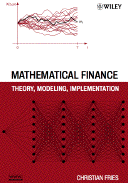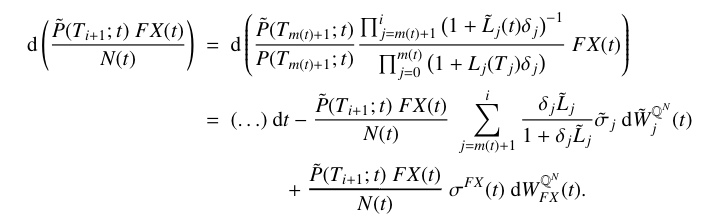Mathematical Finance: Theory, Modeling, Implementation

Version 1.5.12 = First Edition, First Printing.
Version 1.6.11 = First Edition, Second Printing.

Mistakes? We don't make mistakes.
Bill - Department of Works.
Brazil.

# Feedback

Please contact me if you find an error or have a suggestion for an improvement. Please send your feedback to email@christian-fries.de, using the subject "Book Mathematical Finance: Bug report".

If you like the book and it's accompanying material you may consider giving it a positive rating or a review, e.g., at amazon.com, amazon.co.uk, amazon.de, amazon.at, amazon.fr, amazon.jp, Barnes & Noble.com. Thx.

# Errata

The following list gives some of the known errors. The version number indicates which version is affected. You will find the version number of your book on Page v. Last update of this page: 14.02.2017.

Location Description Version Status
Chapter 2, page 41

On page 41, in the 3rd equation replace 2 √(π) by √(2 π).

1.5.12 fixed in 1.6.14
Chapter 3, page 68

In line 5, in the second integrability condition, it should be φ in place of θ (both, θ and φ have to satisfy the integrability condition).

1.5.12 fixed in 1.6.8
Chapter 7, page 104

On page 104, in equation (7.11) add + h.o.t. (higher order terms) to the end of the equation.

1.5.12 fixed in 1.8.7
Chapter 7, page 106

On page 106, in the 6th line from the bottom replace k≠0 by i≠0.

On page 106, in the 3rd line from the bottom replace S0 by S0(t).

1.5.12 fixed in 1.7.4
Chapter 7, page 107

On page 107, in the 10th line from the bottom replace σ2 by σ2(T-t).

On page 107, in the 6th line from the bottom replace σ2 by σ2(T-tk).

On page 107, in the 6th line from the bottom replace φ(tk-1) by φ0(tk-1) (the coefficient of N(tk)).

1.5.12 fixed in 1.7.4
Chapter 7, page 111

On page 111, the formulas for φ0 and φ1 are the corresponding formulas for the delta hedge. The additional correction term of the delta-gamma hedge ψ1 is missing. Download a corrected version of page 111 (pdf).

1.5.12 fixed in 1.7.4
Chapter 12, page 173, Theorem 176

In Theorem 176 a prerequisite is missing. It is required that f is monotonely increasing.

Note: If S is a martingale then this requirement is not necessary. If S is a super-martingale then the corresponding result holds for f being a monotonely decreasing convex function.

1.5.12 fixed in 1.6.4
Chapter 18

Some figures Chapter 18 use a light gray which is a bit hard to distinguish from the background. Solution: You may download a collection of the figures in PDF, see the Mathematical Finance Picture Book.

1.5.12 open, but solution exists
Chapter 19, page 316, line 12

In line 12 on page 316 the index of the swap period is wrong. It is il(k), il(k)+1 and not lk, lk+1. The text in parentheses should read:

...(the index l(k) marks the first swap period [Til(k),Til(k)+1] which contains the forward rate period [Tk,Tk+1])...

1.5.12 fixed in 1.5.17
Chapter 19, page 304

In line 2 the word not has to be deleted. The sentence should read: neither the numéraire N(t) of the terminal measure nor the numéraire of the spot measure is fully described by the processes Li(t).

1.5.12 fixed in 1.6.11
Chapter 19, page 318

In the first equation (the integrated quadratic covariation of the (log)swap rate processes) the first dt has to be deleted.

1.5.12 fixed in 1.6.6
Chapter 24, page 358

On page 358, in the 3rd line from the bottom delete the term ½ t2. The equation should read fT1(t) = fT1(0) + f0(T1 + t) − f0(t) + σ2 T1 t

1.5.12 fixed in 1.7.2
Chapter 27, page 381

On page 381, in the 9th line from above replace S(t) by S(t)/B(t) twice on the right hand side of the equation.

1.5.12 fixed in 1.7.7
Chapter 27, page 381

On page 381, in the 10th line from above replace &sigmaBS2 by &sigmaBS (i.e., delete the 2).

1.5.12 fixed in 1.7.7
Chapter 27, page 382

On page 382, in the 9th line from above the first opening bracket should be placed before exp, i.e., replace K exp(-rT) (&phi(d-)... by K (exp(-rT) &phi(d-)....

1.5.12 fixed in 1.7.7
Chapter 27, page 386

On page 386, in the 7th line from below replace t by ti.

1.5.12 fixed in 1.7.7
Chapter 27, page 387

On page 387, in the 8th line from below replace &sigmaBS(ti)2 by &sigmaBS(ti)2 ti. Likewise, in the 7th line from below replace &sigmaBS(ti+1)2 by &sigmaBS(ti+1)2 ti+1.

1.5.12 fixed in 1.7.7
Chapter 27, page 392 and 396

In Equation (27.15) a closing bracket is missing. Also in the line after Equation (27.15) and in an equation on page 396. N(Ti+1,x(Ti+1) should read N(Ti+1,x(Ti+1))

1.5.12 fixed in 1.5.16
Chapter 27, page 394

On page 394, in Equation (27.18) the right hand side should be preceded by a minus sign.

1.5.12 fixed in 1.7.7
Chapter 27, page 396

In Equation (27.21) a bracket closes to early. (1+L(Tk))(Tk+1-Tk) should read (1+L(Tk)(Tk+1-Tk))

1.5.12 fixed in 1.7.13
Chapter 27, page 397

In Equation (27.22) a closing bracket is missing. (1+L(Tk;x(Tk))(Tk+1-Tk) should read (1+L(Tk;x(Tk))(Tk+1-Tk))

1.5.12 fixed in 1.7.13
Chapter 27, page 399

In the second line of the equation after Equation (27.23) a bracket is missing. 1+L(Ti,x(Ti))(Ti+1-Ti) N(Ti) should read (1+L(Ti,x(Ti))(Ti+1-Ti)) N(Ti).

1.5.12 fixed in 1.5.15
Chapter 28, page 414

On page 414, the third displaystyle equation starting with P(T;t) = ... should read V(T;t) = ...

1.5.12 fixed in 1.7.14
Chapter 28, page 417

On page 417 equation (28.2) should read

N(Ti) is replaced by N(Ti) P(Ti;0)/Pd(Ti;0)

1.5.12 fixed in 1.7.14
Chapter 29, page 424

The second line in the third displaystyle equation contains typos. The correct equation should read(click to enlarge).

1.5.12 fixed in 1.5.15
Chapter 29, page 426

On page 426, in the last line of the first displaystyle equation the fraction should be inverse and the forward rates are both missing the factor (Ti+1-Ti).

1.5.12 fixed in 1.8.10
Chapter 29, page 427

On page 427, in the equation after "In this case" (line 9-12) a power of -1 is missing four times. The (1+L(Tjj) has to be replaced by (1+L(Tjj)-1 (four times).

1.5.12 fixed in 1.6.13
Chapter 29, page 428

On page 428, the additional closing bracket after Li(Ti) has to be deleted (occurs three times on this page).

1.5.12 fixed in 1.6.11
Chapter 29, page 428

On page 428, in the "Interpretation" of the drift in the expression after the integral a minus sign is missing three times. The average drift is log(1+Li(Ti) (Ti+1-Ti)) / (Ti+1-Ti)

1.5.12 fixed in 1.8.11